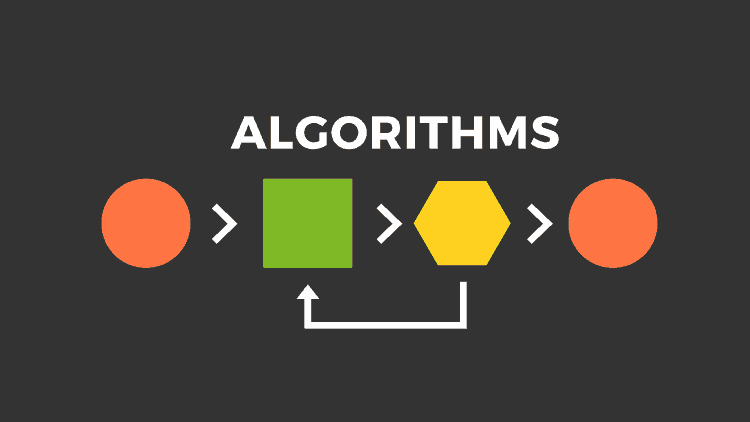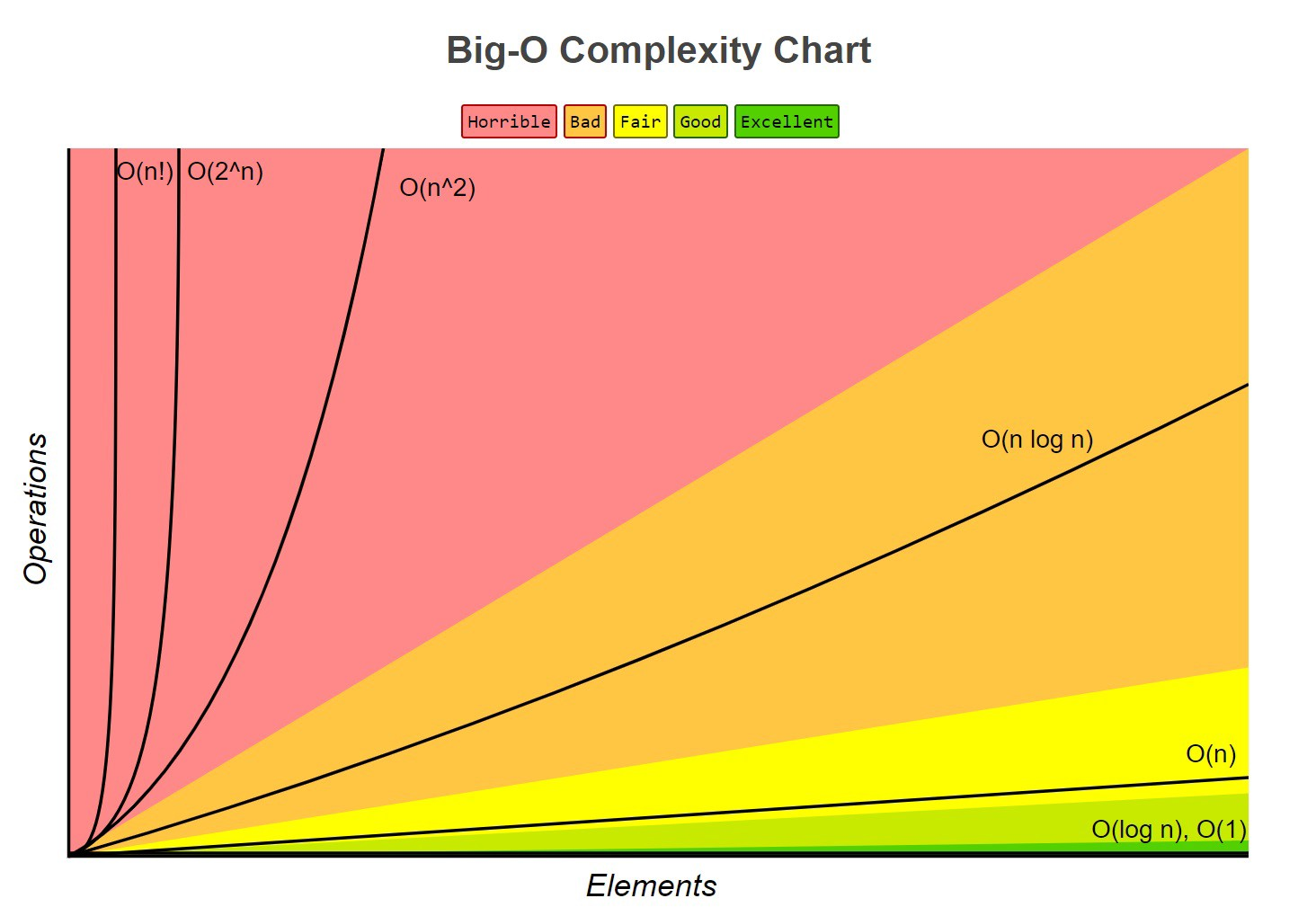# 基礎電腦科學：演算法概要# 前言

1. 放點油
2. 打蛋
3. 如果喜歡蔥花可以加入蔥花，如果喜歡菜脯可以加入菜脯（程式術語：if...else 條件判斷）
4. 放入少許鹽巴
5. 中火快炒，翻五次面（程式術語：for 迴圈）
6. 當看到蛋面呈現金黃色時可以起鍋，結束料理（程式術語：while 迴圈）
7. 好吃的蔥花蛋或菜脯蛋上桌

# 演算法的定義

1. 輸入（Input）：0 或多個輸入
2. 輸出（Output）：至少有一個回傳結果（有可能回傳 0 或是 null）
3. 明確性（Definiteness）：每一個指令步驟必須明確
4. 有限性（Finiteness）：在有限步驟後一定會結束不會進入無窮迴圈
5. 有效性（Effectiveness）：步驟清楚可行，能使用紙筆計算求解

def check_can_vote(age):
if age >= 18:
return True
else:
return False

check_can_vote(20)


# 評估複雜度

## 時間複雜度（Time Complexity）

# 不管 n 輸入為多少，這個程式永遠只會執行一次
def print_num(num):
print(num)

print_num(10)


def sum_number(num):
total = 0
for n in num:
total += num

sum_number(10)


O(nlog(n))## 空間複雜度

def sum_number(num):
total = 0
for n in num:
total += num

sum_number(10)


def sum_number(num):
total = []
for n in num:
total.append(num)

sum_number(10)


# 參考文件

（image via pandorafmsfreecodecamp

@kdchang 文藝型開發者，夢想是做出人們想用的產品和辦一所心目中理想的學校。A Starter & Maker. JavaScript, Python & Arduino/Android lover.:)